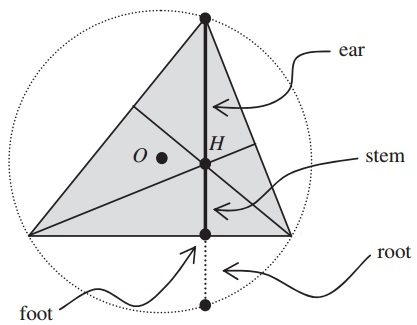The Anatomy of an Altitude

Altitudes have ear, stem, foot, and rootFYI, strangely enough, the midpoint of the ear is called not external acoustic meatus nor ear canal or ear drum but Euler point. No less strange is the fact that the point where the ear is attached to the stem is known not as a skull, or head, or neck, but the orthocenter. As Siamese triples, the three altitudes of a triangle are joined at the orthocenter but, of course, each has its own foot and other anatomical items.

References

1. Jerzy Kocik and Andrzej Solecki, Disentangling a Triangle, Am Math Monthly 116 (March 2009), 228-237A Sample of Optimization Problems

• Mathematicians Like to Optimize
• Reshuffling knights - castle defenders
• Isoperimetric Theorem and Inequality
• Viewing a Statue: the Problem of Regiomontanus
• Fagnano's Problem
• Minimax Principle Demonstration
• Maximum Perimeter Property of the Incircle
• Extremal Problem in a Circular Segment
• Optimization in Four Variables with Two Constraints
• Daniel Dan's Optimization in Three Variables
• Problem in a Special Trapezoid
• Cubic Optimization with Linear Constraints
• Cubic Optimization with Partly Linear Constraints
• Problem M317 from Crux Mathematicorum
• Find the Maximum and Minimum of a Function
• Area of Isosceles Triangle
• Minimum of Cotangents from Saint Petersburg
•